## RS Aggarwal Class 6 Solutions Chapter 10 Ratio, Proportion and Unitary Method Ex 10D

These Solutions are part of RS Aggarwal Solutions Class 6. Here we have given RS Aggarwal Solutions Class 6 Chapter 10 Ratio, Proportion and Unitary Method Ex 10D.

Other Exercises

OBJECTIVE QUESTIONS
Mark against the correct answer in each of the following :

Question 1
Solution:
(d) $$\\ \frac { 92 }{ 115 }$$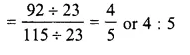Question 2.
Solution:
(a) $$\\ \frac { 57 }{ x }$$
= $$\\ \frac { 51 }{ 85 }$$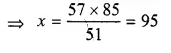Question 3.
Solution:
(a) $$\\ \frac { 25 }{ 35 }$$
= $$\\ \frac { 45 }{ x }$$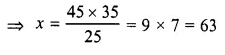Question 4.
Solution:
(c) $$\\ \frac { 4 }{ 5 }$$
= $$\\ \frac { x }{ 35 }$$Question 5.
Solution:
(b) $$\\ \frac { a }{ b }$$
= $$\\ \frac { c }{ d }$$

Question 6.
Solution:
(b) a : b :: b : cQuestion 7.
Solution:
(b) $$\\ \frac { 5 }{ 8 }$$ < $$\\ \frac { 3 }{ 4 }$$ => 4 x 5 < 3 x 8 => 20 < 24

Question 8.
Solution:
Total amount = Rs 760
Ratio A : B = 8 : 11Question 9.
Solution:
(d) largest = $$\\ \frac { 252\times 7 }{ 5+7 }$$
= $$\\ \frac { 252\times 7 }{ 12 }$$
= 21 x 7
= 147

Question 10.
Solution:
(b) largest = $$\\ \frac { 90\times 5 }{ 1+3+5 }$$
= $$\\ \frac { 90\times 5 }{ 9 }$$
= 50 cm

Question 11.
Solution:
(c) total strength of school
largest = $$\\ \frac { 840 }{ 5 }$$ x (12 + 5)
= $$\\ \frac { 840\times 17 }{ 5 }$$
= 168 x 17
= 2856

Question 12.
Solution:
(b) Cost of 12 pens = Rs 138
Cost of 1 pen = Rs $$\\ \frac { 138\times 14 }{ 12 }$$
and cost of 14 pens = Rs 161

Question 13.
Solution:
(b) $$\\ \frac { 24\times 15 }{ 8 }$$
= 45 days

Question 14.
Solution:
(a) $$\\ \frac { 26\times 40 }{ 20 }$$
= 52 men

Question 15.
Solution:
(b) In 6 L of petrol, a car covers = 111 km
In 1 L, it will cover = $$\\ \frac { 111 }{ 6 }$$ km
and in 10 L it will cover = $$\\ \frac { 111\times 10 }{ 6 }$$ km
= 185 km

Question 16.
Solution:
(a) $$\\ \frac { 28\times 550 }{ 700 }$$
= 22 days

Question 17.
Solution:
Ratio in the angles of triangle
= 3 : 1 : 2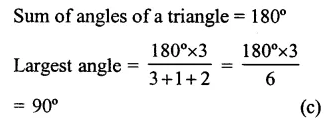Question 18.
Solution:
(b) Ratio in length and breadth of a rectangle = 5 : 4
Width = 36 m
Length = $$\\ \frac { 5 }{ 4 }$$ x 36 m
= 45m

Question 19.
Solution:
Bus covers in 3 hrs = 195 km
It will cover in 1 hr = $$\\ \frac { 195 }{ 3 }$$ = 65 km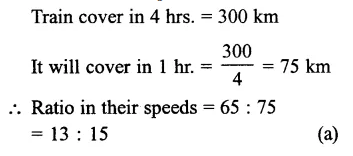Question 20.
Solution:
1 dozen = 12 bars
Cost of 5 bars of soap = Rs.82.50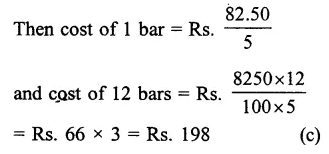Question 21.
Solution:
Total pencils in 30 packets of 8 pencils
= 30 x 8= 240
and total pencils of 25 packets of 12
pencils = 25 x 12 = 300
Now cost of 240 pencils = Rs. 600
Then cost of 1 percent = Rs. $$\\ \frac { 600 }{ 24 }$$
and cost of 300 pencils = Rs. $$\\ \frac { 600\times 300 }{ 240 }$$
= Rs 750 (b)

Question 22.
Solution:
Journey of 75 km costs = Rs 215
Cost of 1 km = Rs $$\\ \frac { 215 }{ 75 }$$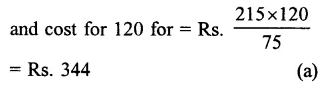Question 23.
Solution:
1st term = 12
2nd term = 21
fourth term = 14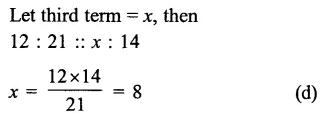Question 24.
Solution:
10 boys dig a patch in = 12 hrs
1 boy will dig it in = 12 x 10 hours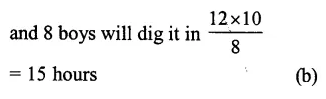Hope given RS Aggarwal Solutions Class 6 Chapter 10 Ratio, Proportion and Unitary Method Ex 10D are helpful to complete your math homework.

If you have any doubts, please comment below. Learn Insta try to provide online math tutoring for you.